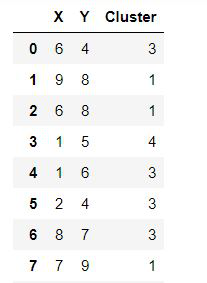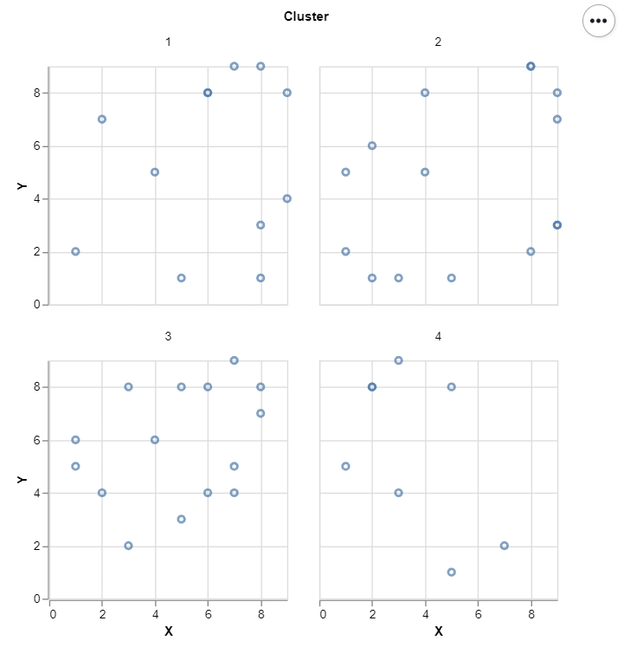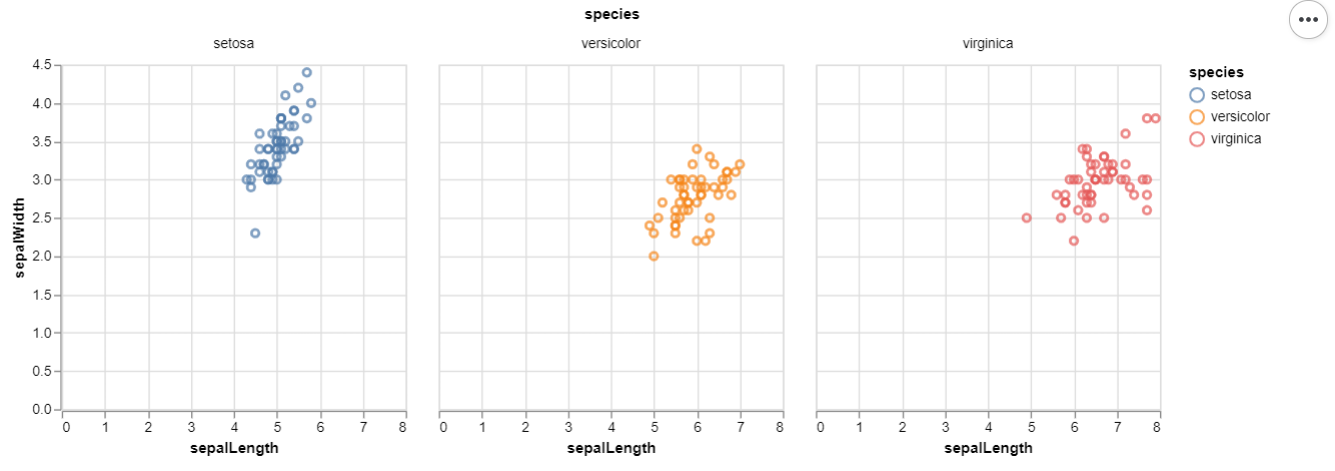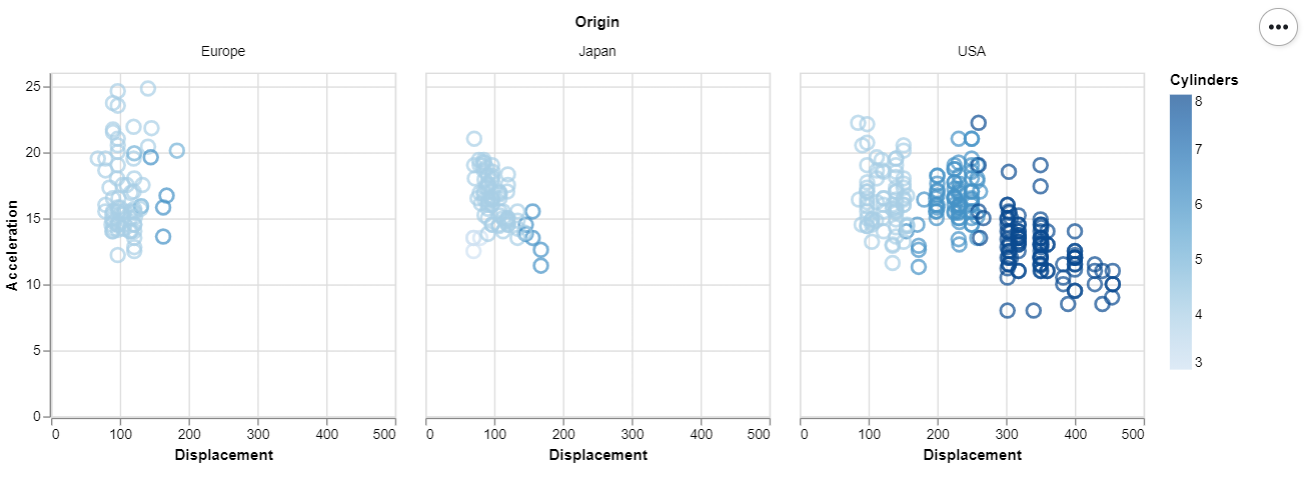GeeksforGeeks App
Open AppBrowser
Continue

# How To Facet a Scatter Plot with Altair?

In this article, we will learn how to Facet a Scatter Plot with Altair. Let’s recall some concepts :

• Altair is a statistical visualization library in Python. It is declarative in nature and is based on Vega and Vega-Lite visualization grammars. It is fast becoming the first choice of people looking for a quick and efficient way to visualize datasets. If you have used imperative visualization libraries like matplotlib, you will be able to rightly appreciate the capabilities of Altair.
• A scatter plot (also called a scatterplot, scatter graph, scatter chart, scattergram, or scatter diagram) is a type of plot or mathematical diagram using Cartesian coordinates to display values for typically two variables for a set of data.

Here, we are making the scatter plot using Altair library. For this, we use Chart() function in Altair to load the data and then use the mark_point() function to make a scatter plot. We then use the aesthetics x and y-axis to encode() function. After making this scatter plot we will facet it with a grouped column values such as clusters.

### Steps Needed

1. Import Libraries (Altair).
3. Use Chart() to load data for plot.
4. Use mark_point() to scatter plot.
5. Use encode() for x and y axes.
6. (Optional)Use properties() for setting width and height.
7. Use facet() over scatter plot with clusters.

### Examples

Let’s understand the above-mentioned steps with the help of some examples :

Example 1:

In this example, we draw a simple facet Scatter plot with some dummy data. That is shown below:Below is the implementation:

## Python3

 `# import libraries``import` `altair as alt``import` `pandas as pd``import` `numpy as np``np.random.seed(``1``)`` ` `# create data``df ``=` `pd.DataFrame({``'X'``:np.random.randint(``1``, ``10``, ``50``),``                   ``'Y'``:np.random.randint(``1``, ``10``, ``50``),``                   ``'Cluster'``:np.random.randint(``1``, ``5``, ``50``)})`` ` `# Draw Facet Scatter Plot``alt.Chart(df).mark_point().encode(``    ``x``=``alt.X(``'X'``),``    ``y``=``alt.Y(``'Y'``)``).properties(width ``=` `200``, height ``=` `200``).facet(``    ``'Cluster:N'``,``    ``columns ``=` `2``)`

Output:Example 2 : (Iris Data From Vega Dataset)

## Python3

 `# import libraries``import` `altair as alt``from` `vega_datasets ``import` `data`` ` `# load data``iris ``=` `data.iris()`` ` `# Draw Facet Scatter Plot``alt.Chart(iris).mark_point().encode(``    ``x ``=` `alt.X(``'sepalLength'``),``    ``y ``=` `alt.Y(``'sepalWidth'``),``    ``color ``=` `'species'``).properties(width ``=` `250``, height ``=` `250``).facet(``    ``'species:N'``,``    ``columns ``=` `3``)`

Output:Example 3 : (Cars Data From Vega Dataset)

## Python3

 `# import libraries``import` `altair as alt``from` `vega_datasets ``import` `data`` ` `# load data``cars ``=` `data.cars()`` ` `# Draw Facet Scatter Plot``alt.Chart(cars).mark_point().encode(``    ``x ``=` `alt.X(``'Displacement'``),``    ``y ``=` `alt.Y(``'Acceleration'``),``    ``size ``=` `alt.value(``100``),``    ``color ``=` `'Cylinders'``).properties(width ``=` `250``, height ``=` `250``).facet(``    ``'Origin:N'``,``    ``columns ``=` `3``)`

Output:My Personal Notes arrow_drop_up